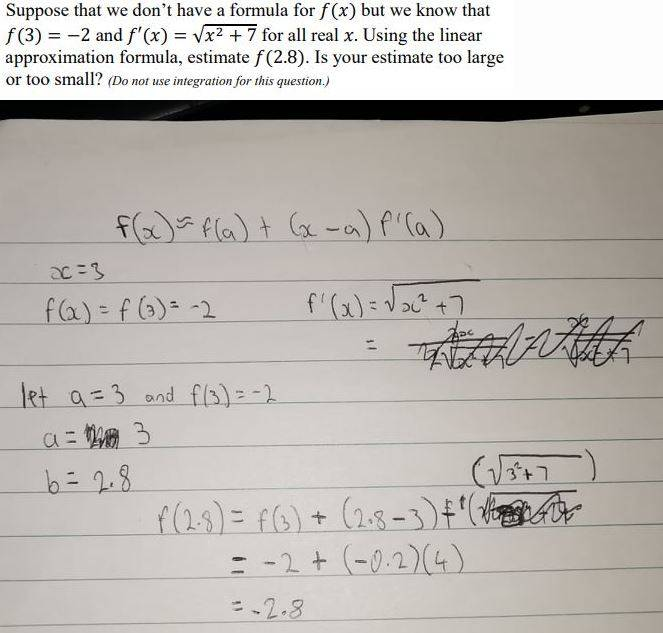# Linear approximation

• MHB
• jaredjjj

#### jaredjjj

Have I solved this linear approximation question correctly?yes, $f(2.8) \approx -2.8$

now, how to determine if that value is an over or under estimate?

yes, $f(2.8) \approx -2.8$

now, how to determine if that value is an over or under estimate?
To answer the second half do I have to use the formula f'(x)=sqrt(x^2+7) which would mean -2.8 would be approximately equal to 3.852 which is the same as 2.8.

To answer the second half do I have to use the formula f'(x)=sqrt(x^2+7) which would mean -2.8 would be approximately equal to 3.852 which is the same as 2.8.

?

The linear approximation is a line tangent to $f(x)$ at the point $(3,-2)$.

What does the sign of $f''(3)$ tell you about the behavior of $f(x)$ at $x=3$ ?